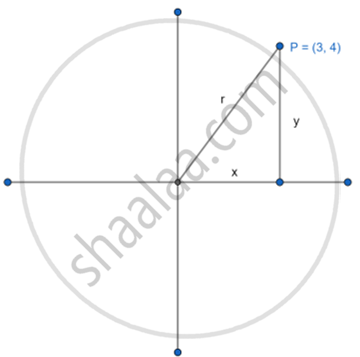# Find the Trigonometric Sine Ratio of an Angle in Standard Position Whose Terminal Arm Passes Through the Point (3, 4). - Geometry

Diagram

Find the trigonometric sine ratio of an angle in a standard position whose terminal arm passes through the point (3, 4).

#### SolutionThe terminal arm passes through (3,4)

x=3 and y=4

r = sqrt("x"^2+"y"^2)

r =sqrt(3^2+4^2) s

r = sqrt(9+16)

r =sqrt25

r = 5

sinθ = "P"/"H" ="y"/"r" = 4/5

Concept: Trigonometric Ratios in Terms of Coordinates of Point
Is there an error in this question or solution?